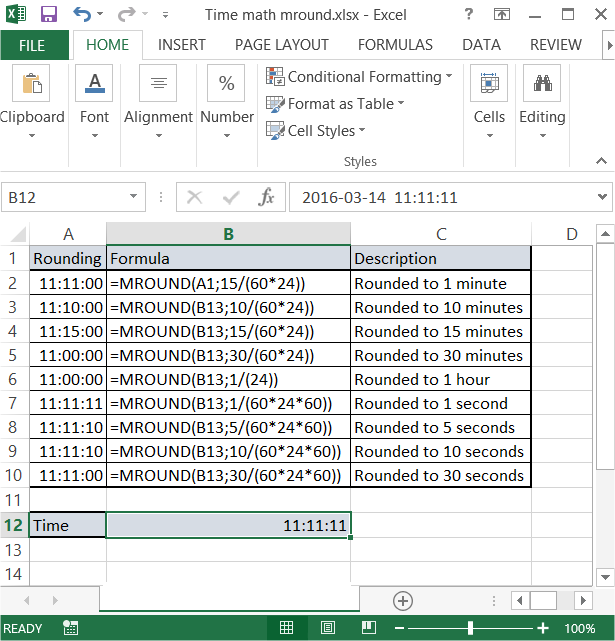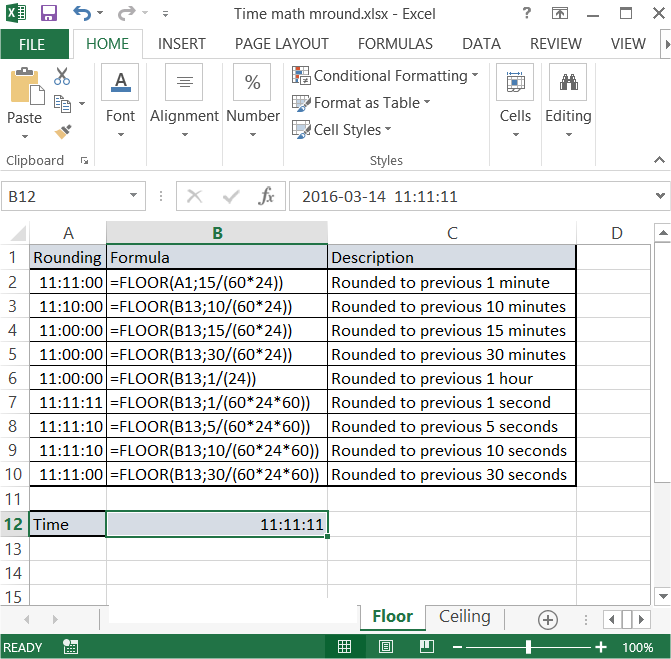### Round To Nearest (Time Math) In Excel

In this Excel tutorial you will learn yourself how to round to nearest time. That kind of time math is useful in spreadsheet where you deal with time.

To round time in Excel just use MROUND function.

Syntax is:

=MROUND(number, multiple)

Number is the time you want to round.

Multiple is the math you will use to do rounding.

In this example there is a 11:11:11 time in B11 cell. There are several rounding’s you can do.

### Rounding to minutes

To round to one minute just use

=MROUND(B11;1/(60*24))

formula. 60 * 24 is the number of minutes in a day.

To round to 15 minutes change 1 to 15 in formula as follows

=MROUND(B11;15/(60*24)) formula.

That’s easy way to round to minutes in Excel.

### Rounding to hours

To round to one hour just use

=MROUND(B11;1/(24)) formula. 24 is the number of hours in a day.

### Rounding to seconds

To round to one second just use

=MROUND(B11;1/(24*60*60)) formula. 24 * 60 * 60 is the number of seconds in a day.

To round to 15 seconds just use

=MROUND(B11;15/(24*60*60)) formula.### Rounding to previous time

To round to previous minute/second/hour just use another function. Instead of MROUND use FLOOR function. Syntax is the same.

Just take a look at below picture and everything should be easy.### Rounding to next time

You can also round to next time. Use CEILING function. Syntax the same.Compare these rounding’s and do some more examples on your own. There are much more possibilities of rounding in Excel.

## Template

```Further reading: